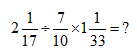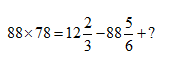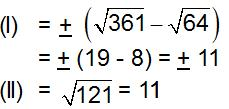Subscribe Now

# Quantitative Aptitude Questions For SBI Clerk : 10 - 06 - 18

Mahendra Guru
Quantitative Aptitude quiz is basically to test your mathematical calculation and approach. It is to find out how fast you can solve a given question with the right methodology of solving the problem. If you know the formulas and short tricks of some important topic in Maths, you will definitely score good marks. So, it is important to know the basic concepts of all the topics so that you can apply the short tricks and solve the question with a new concept in lesser time while giving the quiz. Quantitative Aptitude Quiz helps to evaluate your preparation for banking exam so you can improve your preparation level. Mahendra Guru provides you Quantitative Aptitude Quiz for Bank examination based on the latest pattern so that you can practice on regular basis. It will definitely help you to score good marks in the exam. It is the most important section for all the govt exam like IBPS PO/ Clerk/SO/RRB, RBI, SBI, Insurance, SSC-MTS, CGL, CHSL, State Level and other Competitive exams.

Mahendra Guru provides you a Bank Quiz of Quantitative Aptitude on daily basis to help you in your preparation for Govt jobs. Mahendra Guru also provides you important notes and study material for all subject and test through its website, Mahendra Guru App and YouTube channel apart from it Speed Test Portal. Most of these preparation products are also available for purchase on my shop. You can also visit Mahendras.org to get more information about our endeavour for your success. You can also study in details through our and

Q.1 - What approximate value will come in place of the question mark (?) in the following question?

निम्नलिखित प्रश्न में प्रश्नचिन्ह (?) के स्थान पर लगभग क्या मान आयेगा?1. 25400

2. 28600

3. 29600

4. 29200

5. 24000

Q.2- What approximate value will come in place of the question mark (?) in the following question?

निम्नलिखित प्रश्न में प्रश्नचिन्ह (?) के स्थान पर लगभग क्या मान आयेगा?

77.65 × 981.73 = 8478.73 - 4241.68 + ?

1. 72000

2. 84000

3. 54000

4. 76000

5. 94000

Q.3 - What approximate value will come in place of the question mark (?) in the following question?

निम्नलिखित प्रश्न में प्रश्नचिन्ह (?) के स्थान पर लगभग क्या मान आयेगा?1. 900

2. 700

3. 500

4. 1100

5. 600

Q.4 - What approximate value will come in place of the question mark (?) in the following question?

निम्नलिखित प्रश्न में प्रश्नचिन्ह (?) के स्थान पर लगभग क्या मान आयेगा?1. 3

2. 2

3. 4

4. 4.5

5. None of these

Q.5 - What approximate value will come in place of the question mark (?) in the following question?

निम्नलिखित प्रश्न में प्रश्नचिन्ह (?) के स्थान पर लगभग क्या मान आयेगा?1. 7620

2. 7820

3. 7120

4. 7520

5. 7920

Q.6 In each of the following questions, two equations (I) and (II) are given. You have to solve them

निम्नलिखित प्रश्नों में दो समीकरण (I) और (II) दिये गये हैं दोनों समीकरण हल कीजिए और-1. If (I) > (II)

2. If (I) = (II)

3. If (I) < (II)

4. If (I) > (II)

5. If (I) < (II)

Q.7 In each of the following questions, two equations (I) and (II) are given. You have to solve them

निम्नलिखित प्रश्नों में दो समीकरण (I) और (II) दिये गये हैं दोनों समीकरण हल कीजिए और-

(I) = [(42 ÷ 7) + (63 ÷ 9)] (II) = [(90 - 23) ÷ 5]

1. If (I) > (II)

2. If (I) = (II)

3. If (I) < (II)

4. If (I) > (II)

5. If (I) < (II)

Q.8 In each of the following questions, two equations (I) and (II) are given. You have to solve them and

निम्नलिखित प्रश्नों में दो समीकरण (I) और (II) दिये गये हैं दोनों समीकरण हल कीजिए और-1. If (I) > (II)

2. If (I) = (II)

3. If (I) < (II)

4. If (I) > (II)

5. If (I) < (II)

Q.9 In each of the following questions, two equations (I) and (II) are given. You have to solve them and

निम्नलिखित प्रश्नों में दो समीकरण (I) और (II) दिये गये हैं दोनों समीकरण हल कीजिए और-

(I) = [121 – (43 + 92)] (II) = [ (13)2 × 2 – 325]

1. If (I) > (II)

2. If (I) = (II)

3. If (I) < (II)

4. If (I) > (II)

5. If (I) < (II)

Q.10 In each of the following questions, two equations (I) and (II) are given. You have to solve them.

निम्नलिखित प्रश्नों में दो समीकरण (I) और (II) दिये गये हैं दोनों समीकरण हल कीजिए और-

(I) = [{54 - (5)2} × 3] (II) = [32 × 9 + (3 × 2)]

1. If (I) > (II)

2. If (I) = (II)

3. If (I) < (II)

4. If (I) > (II)

5. If (I) < (II)

Q1. (5)Q2. (1)
78 × 982 = 8479 - 4242 + ?
76596 = 4237 + ?
? = 72359
≈ 72360

Q3. (2)
16 + 787 = 100 + ?
? = 803 - 100 = 703
≈ 700

Q4. (1)? ≈ 3

Q5. (3)
6864 = 13 - 89 + ?
? = 6940
≈ 7120

Q6. (5)Hence/अतः, (I) < (II)

Q7. (3)
(I) = [(42 ÷ 7) + (63 ÷ 9)]
= 6 + 7 = 13
(II) = [(90 – 23) ÷ 5]
= 67 ÷ 5 = 13.4
Hence/अतः, (I) < (II)

Q8. (1)
(I) = 48 + 12 = 60
(II) = 25 + 34 = 59
Hence/अतः, (I) > (II)

Q9. (3)
(I) = 121 – 135 = – 14
(II) = 169 × 2 – 325
= 338 - 325 = 13
Hence/अतः, (I) < (II)

Q10. (2)
(I) = (54 - 25) × 3
= 29 × 3 = 87
(II) = 9 × 9 + 6 = 81 + 6 = 87
Hence/अतः, (I) = (II)